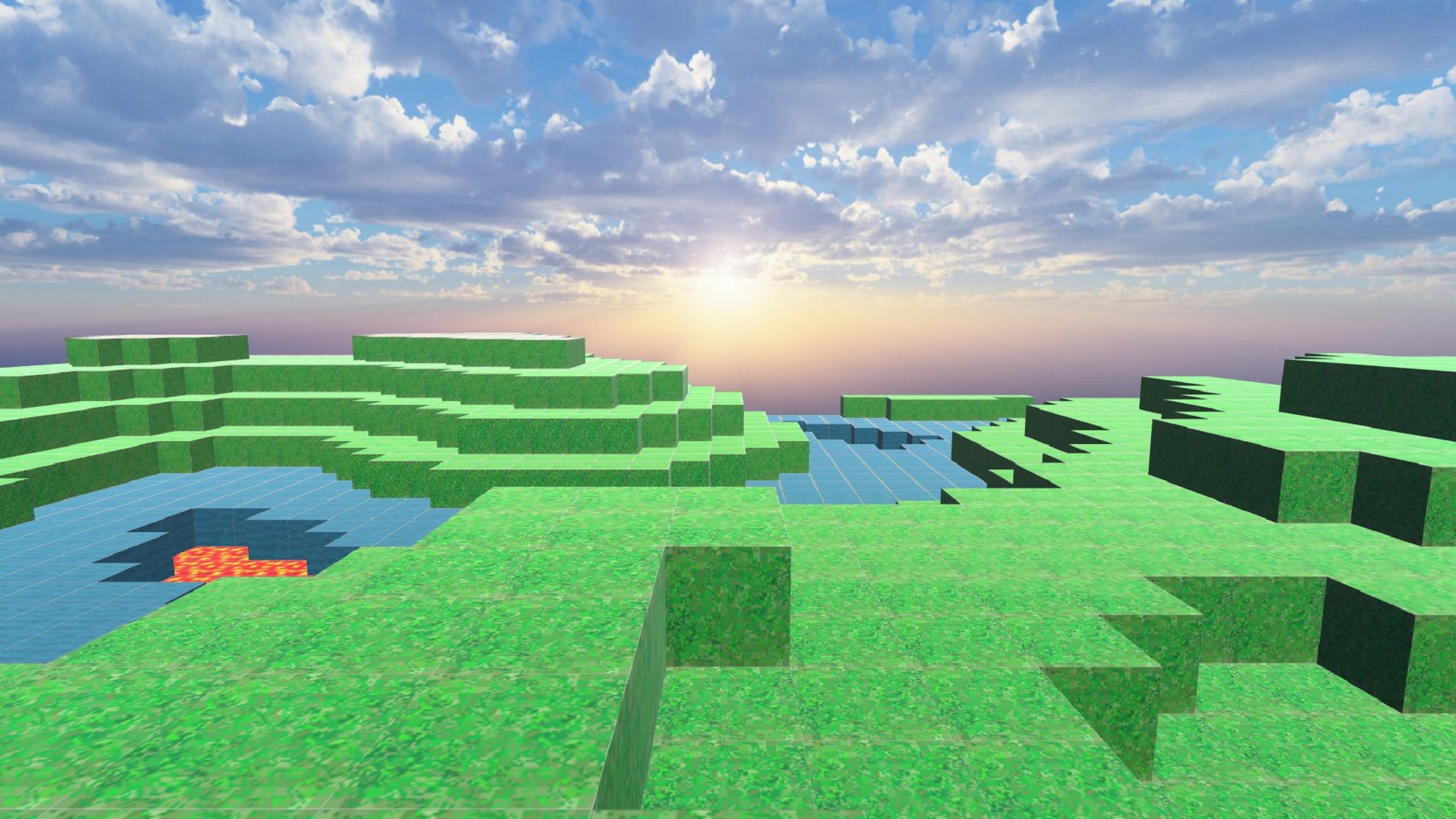# Creating a Minecraft-like landscape and uploading to STYLY -part 2 of 2-

In this article, I will introduce how to create a Minecraft-like landscape and upload it to STYLY.

## Summary of the first part

In the first part, we created the appearance of the landscape, and in this article, I am going to introduce how to upload it to STYLY and finish it up.

• Combining meshes and improving performance
• Save auto-created meshes

I will explain the steps in detail.

## Combining meshes and improving performance

### Preparation

• Adjusting a ground forming object

Adjust “VoxelGround.cs” as in the image below.

```using UnityEngine;

public class VoxelGround : MonoBehaviour
{

private float sizeX = 50f;
private float sizeY = 10f;
private float sizeZ = 50f;
private float sizeW = 17f;

private void Awake()
{
var material = this.GetComponent<MeshRenderer>().material;
for (float x = 0; x < sizeX; x++)
{
for (float z = 0; z < sizeZ; z++)
{
GameObject cube = GameObject.CreatePrimitive(PrimitiveType.Cube);
cube.transform.SetParent(transform);
cube.GetComponent<MeshRenderer>().material = material;

float noise = Mathf.PerlinNoise(x / sizeW, z / sizeW);
float y = Mathf.Round(sizeY * noise);
cube.transform.localPosition = new Vector3(x, y, z);

SetUV(cube);
}
}
Combine();
transform.localPosition = new Vector3(-sizeX / 2, 0, -sizeZ / 2);
}

private void SetUV(GameObject cube)
{
cube.GetComponent<MeshFilter>().mesh.uv = GetBlockUVs(2, 15);

if (cube.transform.position.y > sizeY * 0.3f)
{
cube.GetComponent<MeshFilter>().mesh.uv = GetBlockUVs(0, 15);　//山
}
else if (cube.transform.position.y > sizeY * 0.2f)
{
cube.GetComponent<MeshFilter>().mesh.uv = GetBlockUVs(1, 2);　//水面
}
else if (cube.transform.position.y > sizeY * 0.1f)
{
cube.GetComponent<MeshFilter>().mesh.uv = GetBlockUVs(15, 0);　//溶岩
}
}

private Vector2[] GetBlockUVs(float tileX, float tileY)
{

float pixelSize = 16;
float tilePerc = 1 / pixelSize;

float umin = tilePerc * tileX;
float umax = tilePerc * (tileX + 1);
float vmin = tilePerc * tileY;
float vmax = tilePerc * (tileY + 1);

Vector2[] blockUVs = new Vector2;

//-X
blockUVs = new Vector2(umax, vmax);
blockUVs = new Vector2(umin, vmax);
blockUVs = new Vector2(umax, vmin);
blockUVs = new Vector2(umin, vmin);

//+Y
blockUVs = new Vector2(umin, vmin);
blockUVs = new Vector2(umax, vmin);
blockUVs = new Vector2(umin, vmax);
blockUVs = new Vector2(umax, vmax);

//-Z
blockUVs = new Vector2(umax, vmin);
blockUVs = new Vector2(umin, vmax);
blockUVs = new Vector2(umin, vmin);
blockUVs = new Vector2(umax, vmax);

//+Z
blockUVs = new Vector2(umax, vmin);
blockUVs = new Vector2(umin, vmax);
blockUVs = new Vector2(umin, vmin);
blockUVs = new Vector2(umax, vmax);

//-Y
blockUVs = new Vector2(umax, vmin);
blockUVs = new Vector2(umin, vmax);
blockUVs = new Vector2(umin, vmin);
blockUVs = new Vector2(umax, vmax);

//+X
blockUVs = new Vector2(umin, vmin);
blockUVs = new Vector2(umax, vmin);
blockUVs = new Vector2(umin, vmax);
blockUVs = new Vector2(umax, vmax);

return blockUVs;
}

private void Combine()
{
//Combining Meshes
MeshFilter[] meshFilters = GetComponentsInChildren<MeshFilter>();
CombineInstance[] combine = new CombineInstance[meshFilters.Length];

int i = 0;
while (i < meshFilters.Length)
{
combine[i].mesh = meshFilters[i].sharedMesh;
combine[i].transform = meshFilters[i].transform.localToWorldMatrix;
meshFilters[i].gameObject.SetActive(false);
i++;
}

transform.GetComponent<MeshFilter>().mesh = new Mesh();
transform.GetComponent<MeshFilter>().mesh.CombineMeshes(combine, true);
transform.gameObject.SetActive(true);
}

}```

### Confirming the result

Press the Ctrl + P key, and if a landscape like the one in the image below is created, it is a success.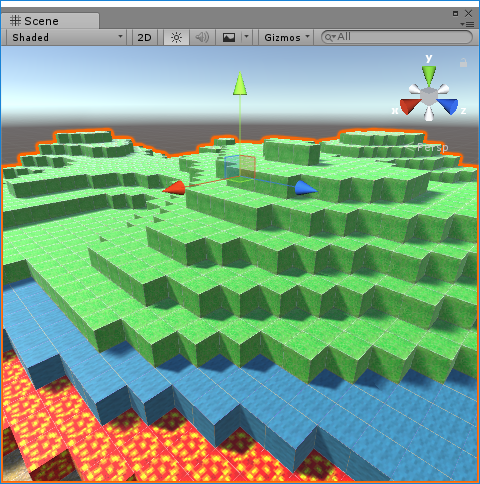Combining 2500 cubes to 1 mesh

### Explanation

```    private void Combine()
{
//Combining meshes
MeshFilter[] meshFilters = GetComponentsInChildren<MeshFilter>();              //The cube mesh created below Ground object
CombineInstance[] combine = new CombineInstance[meshFilters.Length];           //Store the combined meshes in this array

int i = 0;
while (i < meshFilters.Length)
{
combine[i].mesh = meshFilters[i].sharedMesh;                               //Copy the meshes from each cube and copy it to the combined mesh
combine[i].transform = meshFilters[i].transform.localToWorldMatrix;        //Copy the position for each cube and copy it to the combined mesh
meshFilters[i].gameObject.SetActive(false);                                //Nulify the cube objects that are no longer needed
i++;
}

transform.GetComponent<MeshFilter>().mesh = new Mesh();                        //Create a new mesh for the Ground object
transform.GetComponent<MeshFilter>().mesh.CombineMeshes(combine, true);        //Combining the meshes
transform.gameObject.SetActive(true);                                          //Enable the Ground object
}```

We combine the 2500 cubes into 1 mesh here.

The details of the implementation are explained in the comments.

By using the above script, we merge the 2500 cube objects to 1 object.

## Save auto-created meshes

### Preparation

• Introducing the MeshSaver

Implement a tool called MeshSaver from the repository below.

Unity-MeshSaver

The implementation is completing from the following 3 steps

1. Create a folder named “Editor” in the script folder. (CAUTION: If you name the folder a different name, the upload to STYLY will fail)
2. Create a script named MeshSaverEditor in the Editor file
3. Copy and paste the source code from the below URL

MeshSaverEditor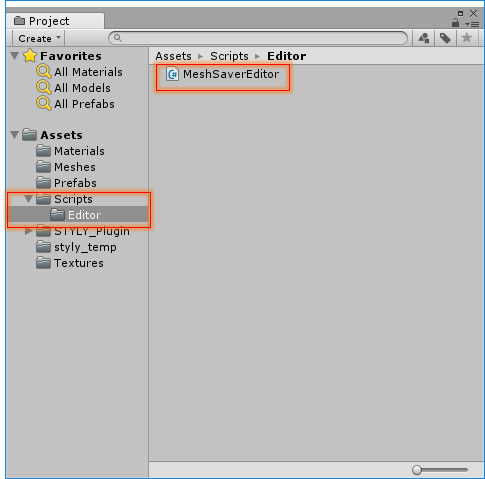Implementing the MeshSave

;

• Saving the Mesh

Press the Ctrl + P key, create a landscape in the Unity editor, and select the landscape object.Select the landscape

Select the “Save Mesh” option in the “Ground (Mesh Filter)” component of the selected object.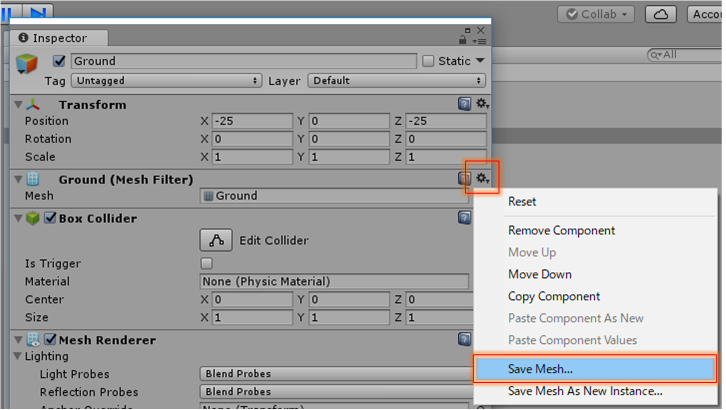Select the designation to save the mesh. ( In the sample, I saved it as Ground.Asset )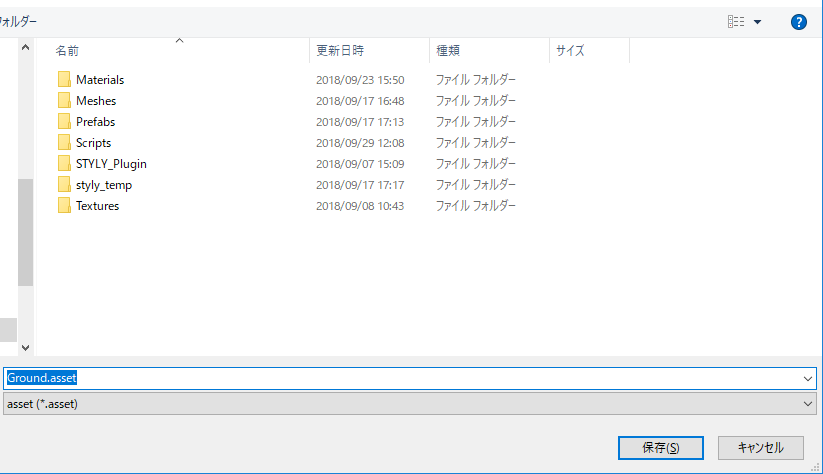Selecting the designation

### Confirming the result

Stop the Unity editor for a moment, select the Ground object, and click the mesh property select button of the Cube ( Mesh Filter ).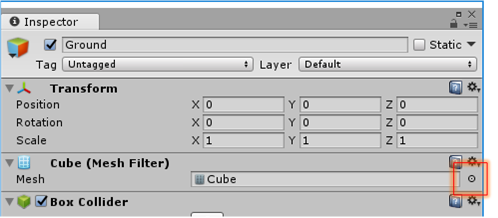Opening the Mesh property

By selecting the “Ground.Asset” you just saved, a landscape mesh will appear in the Unity editor.

If the mesh is shown even if the Unity editor is stopped, it is a success.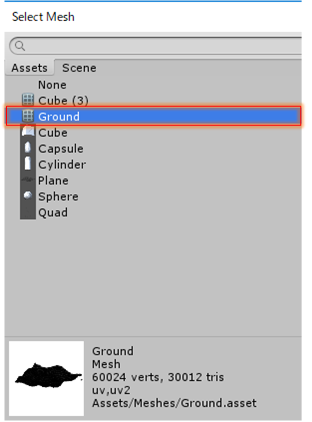Selecting the landscape mesh

### Preparation

• Creating a game object to use for the prefab

Create a cube to make the prefab from.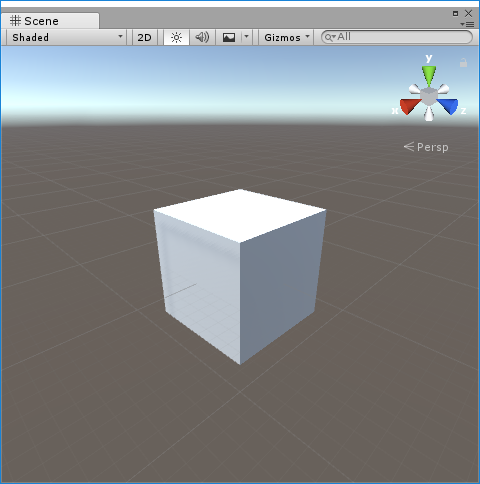The cube to use for the prefab

Select the created cube, and perform the next two steps.

1. Change the Mesh property of Cube (Mesh Filter) to “Ground”
2. Change the Material property of Mesh Rendrerer to “terrain 6.26.56 PM”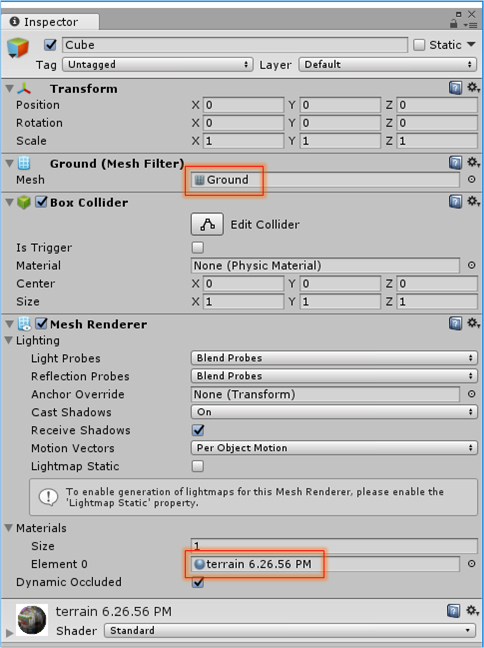Change the mesh and material

• Make a prefab from the game object

Drag and drop the object to the prefabs folder.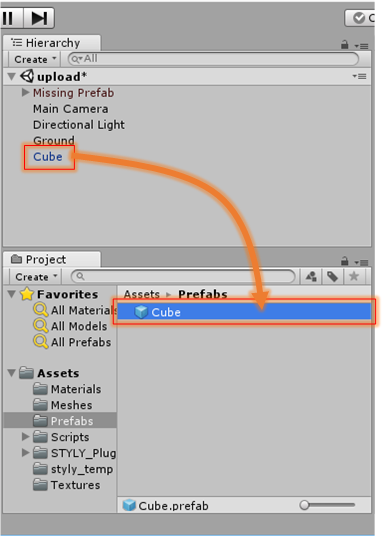Make the cube into a Prefab

In order to upload the prefab to STYLY, you need to set an account and install the plugin.

For the details, refer to the following URL.

How to upload assets from Unity to STYLY

### Confirm the results

You can select your uploaded prefabs from the “MyModels” folder in the STYLY editor.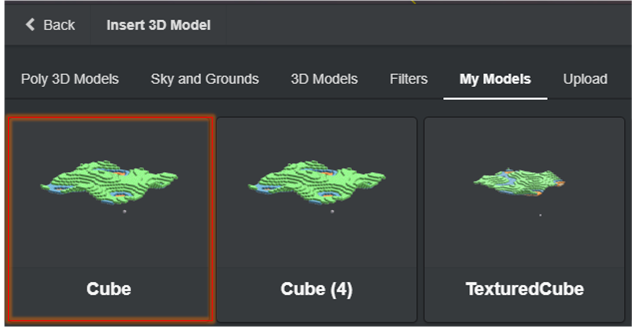Then, change the default landscape display to OFF, and adjust the cube display positions, and you’re done.

If you create the same landscape as in the sample, set X:-25、Y:-7.5、Z:-25 and the landscape will be placed as in the image below.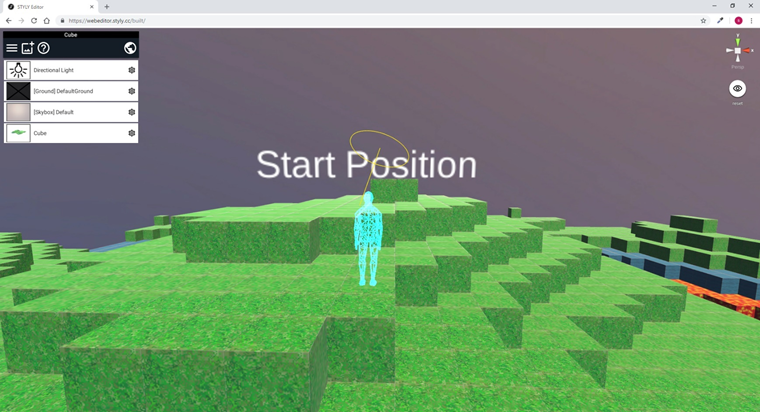The Minecraft-like landscape imported to STYLY

## Conclusion

That will be all for the tutorial for Creating a Minecraft-like landscape and uploading to STYLY.

You can add details to the scene by drawing caves, animals, plants using TiltBrush, or creating fireworks, exploding balls, adding assets. By combining assets from previous articles, you can create an even interesting world.

This is not an official product of Minecraft. This product is not approved from Mojang, and is not related to Mojang.